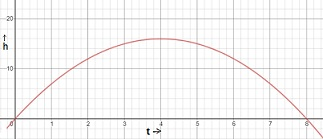# A ball is thrown in the air. The path of the ball is represented by the equation h = -t2 + 8t ....

## Question:

A ball is thrown in the air. The path of the ball is represented by the equation {eq}h = -t2 + 8t {/eq}. Graph the equation over the interval {eq}0 < t < 8 {/eq}.

a. Find the maximum height of the ball.

b. Find how long the ball was in the air.

c. Find the range of heights the ball travels.

## Finding maximum of a function:

Let f(x) be a function of x.

Now, if {eq}f'(x)=0, \: when\: x=x_0\\ and \: f''(x)<0, \:when\: x=x_0, {/eq}

then f(x) is minimum at {eq}x=x_0 {/eq}

and the minimum value is {eq}f(x_0) {/eq}

The path of the ball is represented by the equation {eq}h = -t^2 + 8t ,\: 0 < t < 8 {/eq}.

Clearly, the equation will be in the form of a parabola.

The graph is shown belowa. Clearly from the graph it can be seen that the maximum height of the ball is at h=16.

It can also be found mathematically.

{eq}h=-t^2+8t\\ \text{Differentiating with respect to t,} h'=-2t+8\\ \text{Now, equating with } h'=0,\\ -2t+8=0\\ or,\:t=4\\ \text{Differentiating } h'=-2t+8\: \text{with respect to t},\\ h''=-2<0\\ \text{Hence,}\: h=-t^2+8t\: \text{is maximum at } t=4\\ \text{Therefore the minimum value of h is }\\ h=-(4)^2+(8\times 4)\\ =16 {/eq}

b. From the graph it can be seen that when t=8, the value of h is 0 i.e. the ball falls in the ground.

Hence it can be said that the ball was in the air is for 8 mins.

It can also be found from the equation.

{eq}h=-t^2+8t\\ Now\: if\: h=0, \: then\\ -t^2+8t=0\\ \Rightarrow t=0\:or \:8\\ {/eq}

Now t=0 is not possible because it means when the time was 0, so the only option will be t=8.

c. The maximum height of 16, the ball attains when t=4.

Since the value of the height cannot be negative and the height of the ball will be 0 at the starting point when the ball is thrown.

Hence the minimum value of the height is 0.

Therefore the range of heights the ball travels is from 0 to 16.